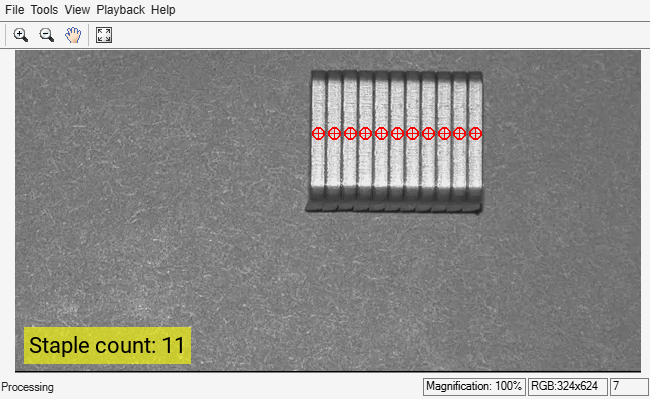# Object Counting

This example shows how to use morphological operations to count objects in a video stream.

### Introduction

The input video stream contains images of staples. In this example, you use the top-hat morphological operation to remove uneven illumination, and the opening morphological operation to remove gaps between the staples. You then convert the images to binary, using a different threshold for each frame. Once this threshold is applied, you count the number of staples and calculate the centroid of each staple.

### Initialization

Use these next sections of code to initialize the required variables and System objects.

Create a System object to read video from avi file.

```filename = 'staples.mp4'; hVideoSrc = VideoReader(filename);```

Create a blob analysis System object to count the staples and find their centroids.

```hBlob = vision.BlobAnalysis( ... 'AreaOutputPort', false, ... 'BoundingBoxOutputPort', false, ... 'OutputDataType', 'single');```

Create a System object to display the output video.

```hVideoOut = vision.VideoPlayer('Name', 'Counted Staples'); hVideoOut.Position(3:4) = [650 350];```

### Stream Processing Loop

Here you call the processing loop to count the staples in the input video. This loop uses the System objects you instantiated.

The loop is stopped when you reach the end of the input file, which is detected by the BinaryFileReader System object.

```while hasFrame(hVideoSrc) I = im2gray(readFrame(hVideoSrc)); Im = imtophat(I, strel('square',18)); Im = imopen(Im, strel('rect',[15 3])); th = multithresh(Im); % Determine threshold using Otsu's method BW = Im > th; Centroids = step(hBlob, BW); % Blob Analysis StaplesCount = int32(size(Centroids,1)); txt = sprintf('Staple count: %d', StaplesCount); It = insertText(I,[10 280],txt,'FontSize',22); % Display staples count Centroids(:, 2) = Centroids(1,2); % Align markers horizontally It = insertMarker(It, Centroids, 'o', 'Size', 6, 'Color', 'r'); It = insertMarker(It, Centroids, 'o', 'Size', 5, 'Color', 'r'); It = insertMarker(It, Centroids, '+', 'Size', 5, 'Color', 'r'); step(hVideoOut, It); end```### Summary

The output video shows the individual staples marked with a circle and plus sign. It also displays the number of staples that appear in each frame.